# **DO IT AS PYTHON PLEASE** The Trifid Cipher General Problem Description The Trifid cipher (not to be confused with the...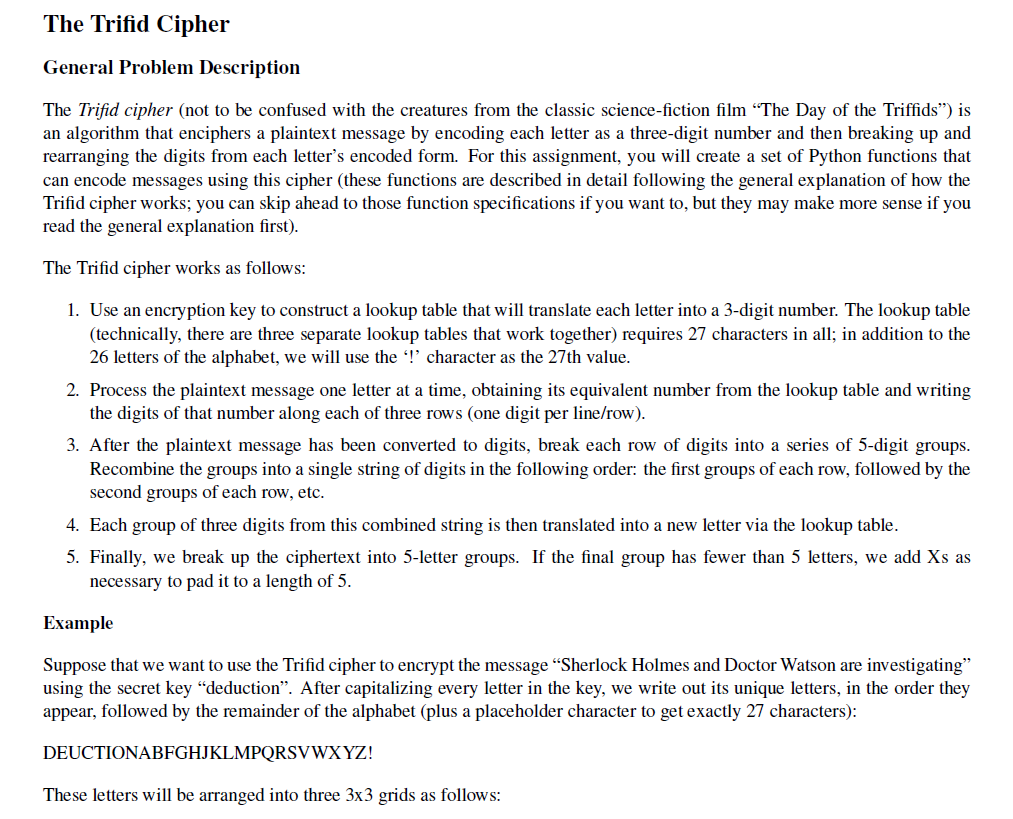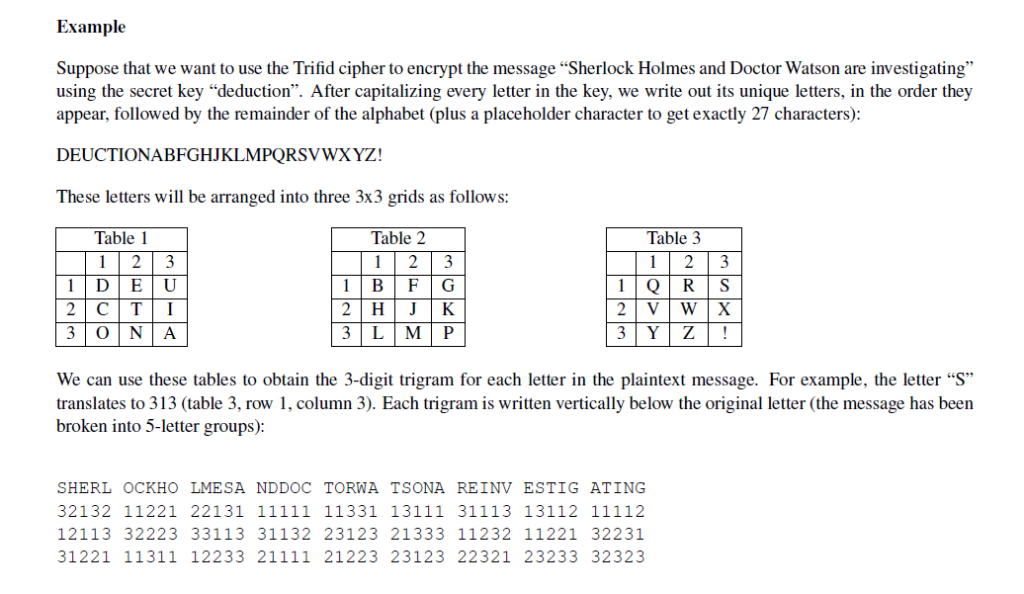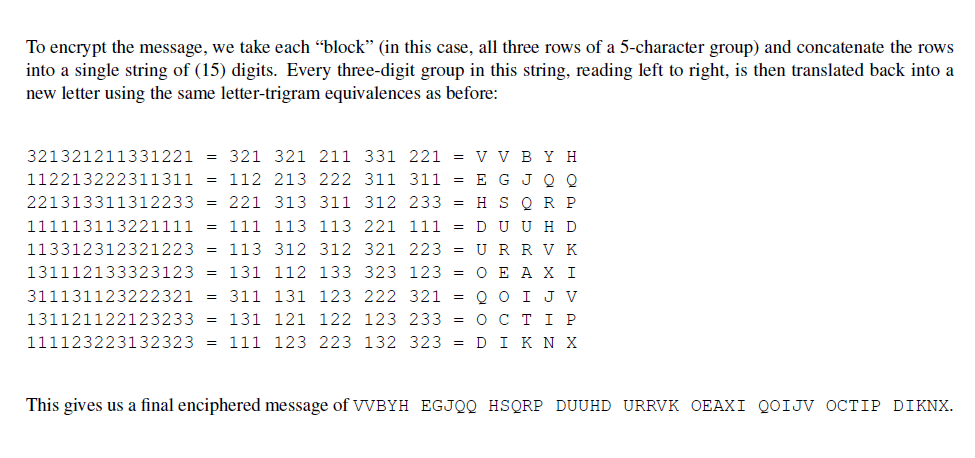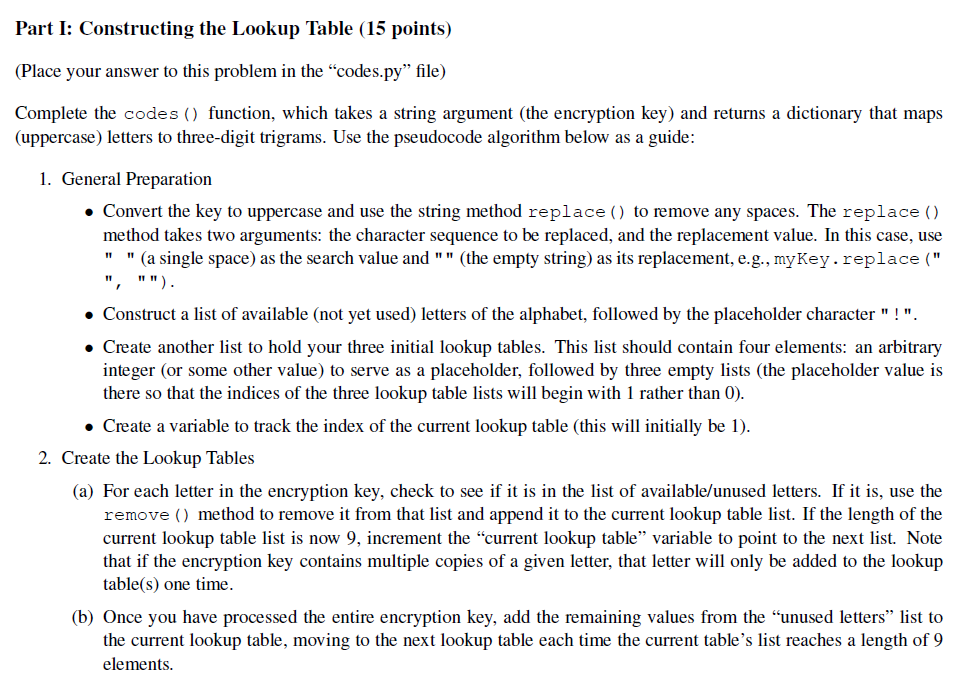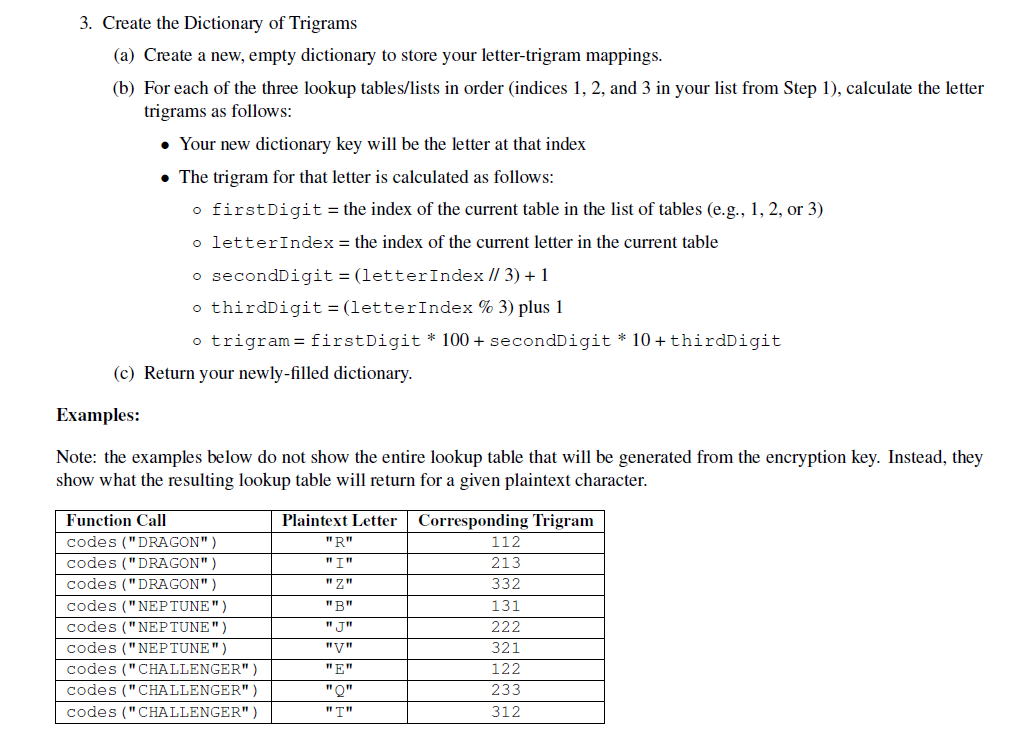The Trifid Cipher General Problem Description The Trifid cipher (not to be confused with the creatures from the classic science-fiction film "The Day of the Triffids") is an algorithm that enciphers a plaintext message by encoding each letter as a three-digit number and then breaking up and rearranging the digits from each letter's encoded form. For this assignment, you will create a set of Python functions that can encode messages using this cipher (these functions are described in detail following the general explanation of how the Trifid cipher works; you can skip ahead to those function specifications if you want to, but they may make more sense if you read the general explanation first) The Trifid cipher works as follows 1. Use an encryption key to construct a lookup table that will translate each letter into a 3-digit number. The lookup table (technically, there are three separate lookup tables that work together) requires 27 characters in all; in addition to the 26 letters of the alphabet, we will use the '!' character as the 27th value 2. Process the plaintext message one letter at a time, obtaining its equivalent number from the lookup table and writing the digits of that number along each of three rows (one digit per line/row) 3. After the plaintext message has been converted to digits, break each row of digits into a series of 5-digit groups Recombine the groups into a single string of digits in the following order: the first groups of each row, followed by the second groups of each row, etc 4. Each group of three digits from this combined string is then translated into a new letter via the lookup table 5. Finally, we break up the ciphertext into 5-letter groups. If the final group has fewer than 5 letters, we add Xs as necessary to pad it to a length of 5 Example Suppose that we want to use the Trifid cipher to encrypt the message "Sherlock Holmes and Doctor Watson are investigating" using the secret key "deduction". After capitalizing every letter in the key, we write out its unique letters, in the order they appear, followed by the remainder of the alphabet (plus a placeholder character to get exactly 27 characters) DEUCTIONABFGHJKLMPQRSVWXYZ! These letters will be arranged into three 3x3 grids as follows
Example Suppose that we want to use the Trifid cipher to encrypt the message "Sherlock Holmes and Doctor Watson are investigating using the secret key "deduction". After capitalizing every letter in the key, we write out its unique letters, in the order they appear, followed by the remainder of the alphabet (plus a placeholder character to get exactly 27 characters) DEUCTIONABFGHJKLMPQRSVWXYZ! These letters will be arranged into three 3x3 grids as follows: Table 1 Table 2 Table 3 12 3 We can use these tables to obtain the 3-digit trigram for each letter in the plaintext message. For example, the letter "S translates to 313 (table 3, row 1, column 3). Each trigram is written vertically below the original letter (the message has beein broken into 5-letter groups) SHERL OCKHO LMESA NDDOC TORWA TSONA REINV ESTIG ATING 12113 32223 33113 31132 23123 21333 11232 11221 32231 31221 11311 12233 21111 21223 23123 22321 23233 32323
To encrypt the message, we take each "block" (in this case, all three rows of a 5-character group) and concatenate the rows into a single string of (15) digits. Every three-digit group in this string, reading left to right, is then translated back into a new letter using the same letter-trigram equivalences as before: 321321211331221 = 321 321 211 331 221 = VV B Y H 112213222311311 = 112 213 222 311 311 = E G J Q Q 221313311312233-221 313 311 312 233 -HSQR P 113312312321223 113 312 312 321 223 U RRV 131112133323123 = 131 112 133 323 123 = O E AX ! 311131123222321 311 131 123 222 321 -Q 0 1 J ν 131121122123233 = 131 121 122 123 233 = О С Т 1 P 111123223132323 = 111 123 223 132 323 = D I K N X This gives us a final enciphered message of VVBYH EGJQQ HSQRP DUUHD URRVK OEAXI QOIJV OCTIP DIKNX.
Part I: Constructing the Lookup Table (15 points) (Place your answer to this problem in the "codes.ру" file) Complete the codesO function, which takes a string argument (the encryption key) and returns a dictionary that maps (uppercase) letters to three-digit trigrams. Use the pseudocode algorithm below as a guide: 1. General Preparation Convert the key to uppercase and use the string method replace ) to remove any spaces. The replace () method takes two arguments: the character sequence to be replaced, and the replacement value. In this case, use "" (a single space) as the search value and "" (the empty string) as its replacement, e.g.,myKey.replace (" Construct a list of available (not yet used) letters of the alphabet, followed by the placeholder character"! Create another list to hold your three initial lookup tables. This list should contain four elements: an arbitrary integer (or some other value) to serve as a placeholder, followed by three empty lists (the placeholder value is there so that the indices of the three lookup table lists will begin with 1 rather than 0). . Create a variable to track the index of the current lookup table (this will initially be 1) 2. Create the Lookup Tables (a) For each letter in the encryption key, check to see if it is in the list of available/unused letters. If it is, use the remove ) method to remove it from that list and append it to the current lookup table list. If the length of the current lookup table list is now 9, increment the "current lookup table" variable to point to the next list. Note that if the encryption key contains multiple copies of a given letter, that letter will only be added to the lookup table(s) one time Once you have processed the entire encryption key, add the remaining values from the "unused letters" li the current lookup table, moving to the next lookup table each time the current table's list reaches a length of 9 elements.
3. Create the Dictionary of Trigrams (a) Create a new, empty dictionary to store your letter-trigram mappings. (b) For each of the three lookup tables/lists in order (indices 1, 2, and 3 in your list from Step 1), calculate the letter trigrams as follows » Your new dictionary key will be the letter at that index The trigram for that letter is calculated as follows o first Digit the index of the current table in the list of tables (e.g., 1, 2, or 3) the index of the current letter in the current table o letter Index o secondDigit = (1etter Index 113) + 1 thirdDigit-(let terindex % 3) plus 1 o trigram- firstDigit 100+ secondDigit *10+thirdDigit (c) Return your newly-filled dictionary Examples: Note: the examples below do not show the entire lookup table that will be generated from the encryption key. Instead, they show what the resulting lookup table will return for a given plaintext character Function Call Plaintext LetterCorresponding Trigram codes (" DRAGON") 112 codes (" DRAGON") 213 codes (" DRAGON") 332 codes ("NEPTUNE") 131 II RIn codes (" NEPTUNE") I TIT 321 codes ("NEPTUNE") codes ("CHALLENGER" ) 122 nFn codes ("CHALLENGER" ) 233 codes ("CHALLENGER") 312 ITI

Task : Constructing The Lookup Table (complete the codes() function ; )

Python CODE ::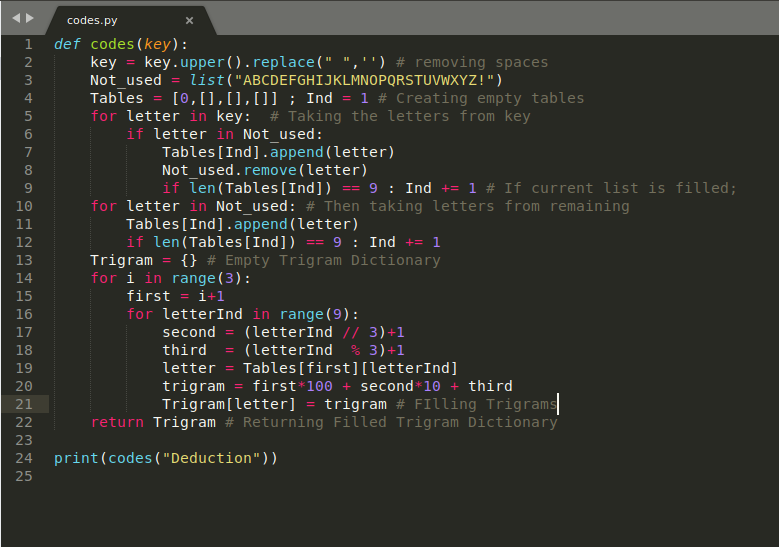Execution (OUTPUT)::..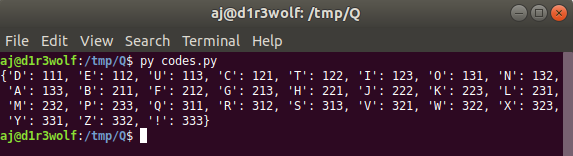The key i have used is : "Deduction"

RAW PYTHON CODE ::

_____________________________ codes.py ____________________________________________________

def codes(key):
key = key.upper().replace(" ",'') # removing spaces
Not_used = list("ABCDEFGHIJKLMNOPQRSTUVWXYZ!")
Tables = [0,[],[],[]] ; Ind = 1 # Creating empty tables
for letter in key:   # Taking the letters from key
if letter in Not_used:
Tables[Ind].append(letter)
Not_used.remove(letter)
if len(Tables[Ind]) == 9 : Ind += 1 # If current list is filled;
for letter in Not_used: # Then taking letters from remaining
Tables[Ind].append(letter)
if len(Tables[Ind]) == 9 : Ind += 1
Trigram = {} # Empty Trigram Dictionary
for i in range(3):
first = i+1
for letterInd in range(9):
second = (letterInd // 3)+1
third = (letterInd % 3)+1
letter = Tables[first][letterInd]
trigram = first*100 + second*10 + third
Trigram[letter] = trigram # FIlling Trigrams
return Trigram # Returning Filled Trigram Dictionary

print(codes("Deduction"))
################################################END#########################################

#### Earn Coin

Coins can be redeemed for fabulous gifts.

Similar Homework Help Questions
• ### Creating a Vigenère Cipher Encryption/Decryption in Assembly Language NASM/YASM With these instructions so far we created...

Creating a Vigenère Cipher Encryption/Decryption in Assembly Language NASM/YASM With these instructions so far we created some variables for the data and the outputs for them but are very confused on how to integrate the cipher portion so help would be appreciated. As seen in the picture below we are supposed to use a Vigenere cipher which uses key and plaintext variables in nasm/yasm assembly. The key is extended to the size of the text and then for each letter...

• ### Caesar Cipher v3 Decription Description A Caesar cipher is one of the first and most simple...

Caesar Cipher v3 Decription Description A Caesar cipher is one of the first and most simple encryption methods. It works by shifting all letters in the original message (plaintext) by a certain fixed amount (the amounts represents the encryption key). The resulting encoded text is called ciphertext. Example Key (Shift): 3 Plaintext: Abc Ciphertext: Def Task Your goal is to implement a Caesar cipher program that receives the key and an encrypted paragraph (with uppercase and lowercase letters, punctuations, and...

• ### do the following in python idle 3.5 or higher know nothing on this subject Eile Edit...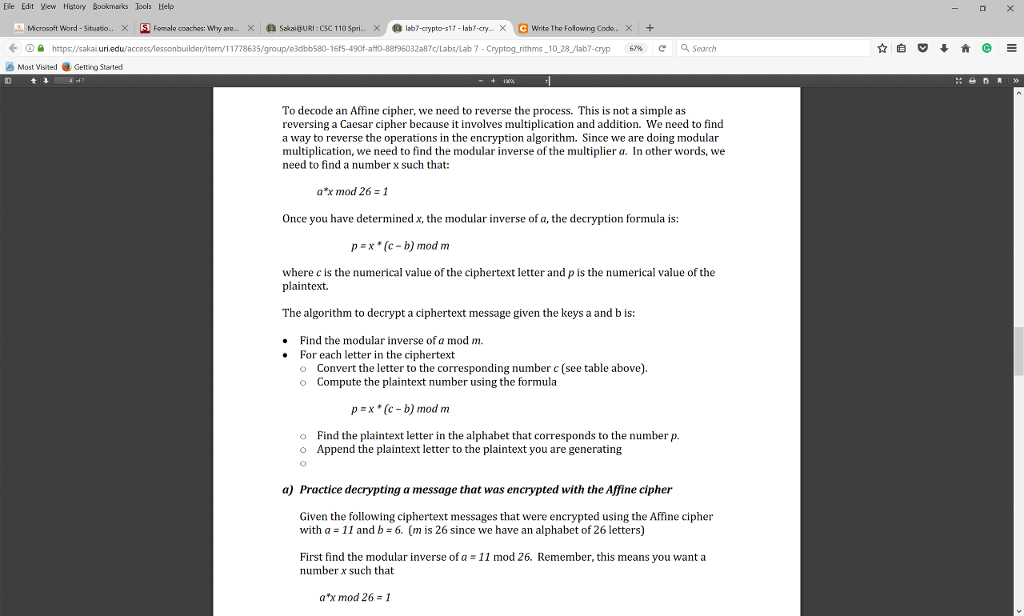do the following in python idle 3.5 or higher know nothing on this subject Eile Edit Yew Hbtory Bookmarks bols Help Microsoft Word-Situatio x S Female coaches: Why are. x a SakaieURI:csc 110 spri.. x O lab7-crypto-s17-labr-cry. x Writer The owing Code. x https sakai. 7-Cryptog rithms -10 28 /lab7-cryp 67k e a Search A Most visited e Getting started To decode an Affine cipher, we need to reverse the process. This is not a simple as reversing a Caesar...

• ### JAVA PROJECT Part 1 - Normalize Text The first thing we will do is normalize the...

JAVA PROJECT Part 1 - Normalize Text The first thing we will do is normalize the input message so that it’s easier to work with. Write a method called normalizeText which does the following: Removes all the spaces from your text Remove any punctuation (. , : ; ’ ” ! ? ( ) ) Turn all lower-case letters into upper-case letters Return the result. The call normalizeText(“This is some \“really\” great. (Text)!?”) should return “THISISSOMEREALLYGREATTEXT” Part 2 - Obfuscation...

• ### Write a program that implements an elementary bit stream cipher. An elementary level bit stream cipher...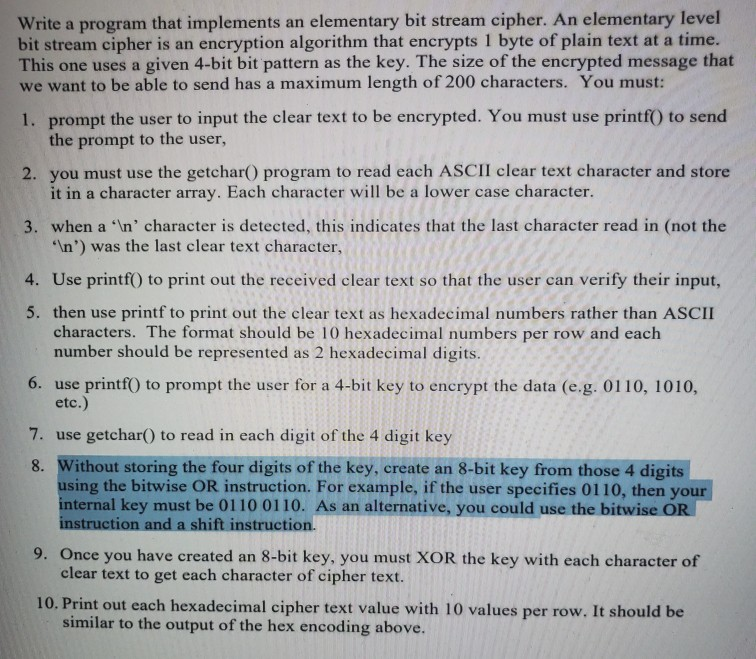Write a program that implements an elementary bit stream cipher. An elementary level bit stream cipher is an encryption algorithm that encrypts 1 byte of plain text at a time. This one uses a given 4-bit bit pattern as the key. The size of the encrypted message that we want to be able to send has a maximum length of 200 characters. You must: 1. prompt the user to input the clear text to be encrypted. You must use printf()...

• ### Python code. NO importing modules. you may not use: contains(), index(), sum(),join(), split(). def column_cipher(plaintext): •...

Python code. NO importing modules. you may not use: contains(), index(), sum(),join(), split(). def column_cipher(plaintext): • Parameter(s): plaintext ----- a string; the message to be encrypted • Return value: A string; the ciphertext after applying the column cipher algorithm • Assumptions: o The standard English alphabet is used: "abcdefghijklmnopqrstuvwxyz" o All strings will be non-empty, entirely lowercase, and only contain valid letters in the alphabet. • How it works: o First, transpose the text into 5 different columns by writing...

• ### Python program Use the provided shift function to create a caesar cipher program. Your program s...

python program Use the provided shift function to create a caesar cipher program. Your program should have a menu to offer the following options: Read a file as current message Save current message Type in a new message Display current message "Encrypt" message Change the shift value For more details, see the comments in the provided code. NO GLOBAL VARIABLES! Complete the program found in assignment.py. You may not change any provided code. You may only complete the sections labeled:#YOUR...

• ### LANGUAGE: PYTHON Write a function called: d_polybius(). The function applies the decryption scheme for the polybius...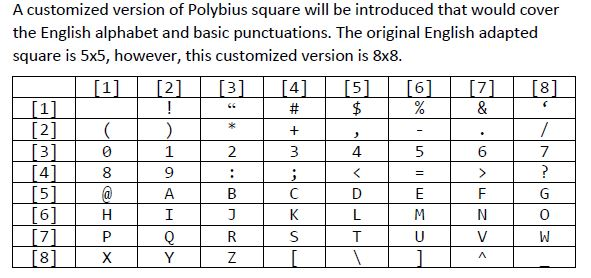LANGUAGE: PYTHON Write a function called: d_polybius(). The function applies the decryption scheme for the polybius cipher scheme above. The start of the function call the get_polybius_square function to get the square as a string. The second scenario when the number of characters is not even, excluding ‘\n’. For instance: “71\n5” is an invalid cipher because there is no way that the last number correspond to a character (we need two numbers). A customized version of Polybius square will be...

• ### 1) Echo the input: First, you should make sure you can write a program and have...

1) Echo the input: First, you should make sure you can write a program and have it compile and run, take input and give output. So to start you should just echo the input. This means you should prompt the user for the plaintext, read it in and then print it back out, with a message such as "this is the plaintext you entered:". [4 points, for writing a working program, echoing the input and submitting the program on the...

• ### For this problem, you will write a program to form a frequency table of the letters...

For this problem, you will write a program to form a frequency table of the letters in a text file, that is, how many times each letter appears in the file In Python 3.7. Such a frequency table might be useful for compressing a text file. Because different letters appear with different frequencies, we can compress a file by using shorter codes for common letters and longer codes for letters that appear less frequently. Dictionaries provide an elegant way to...

Free Homework App